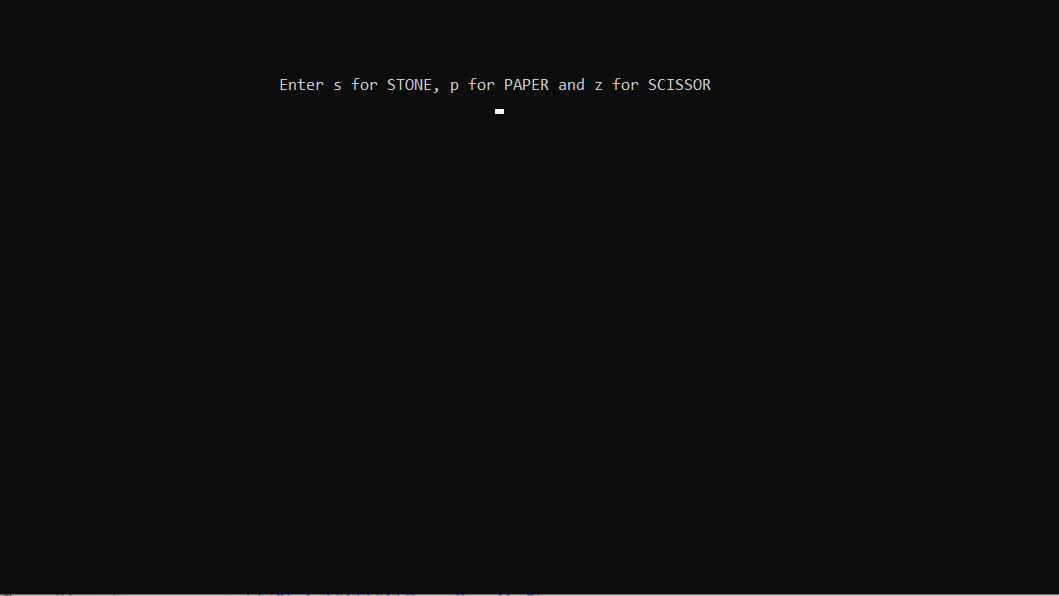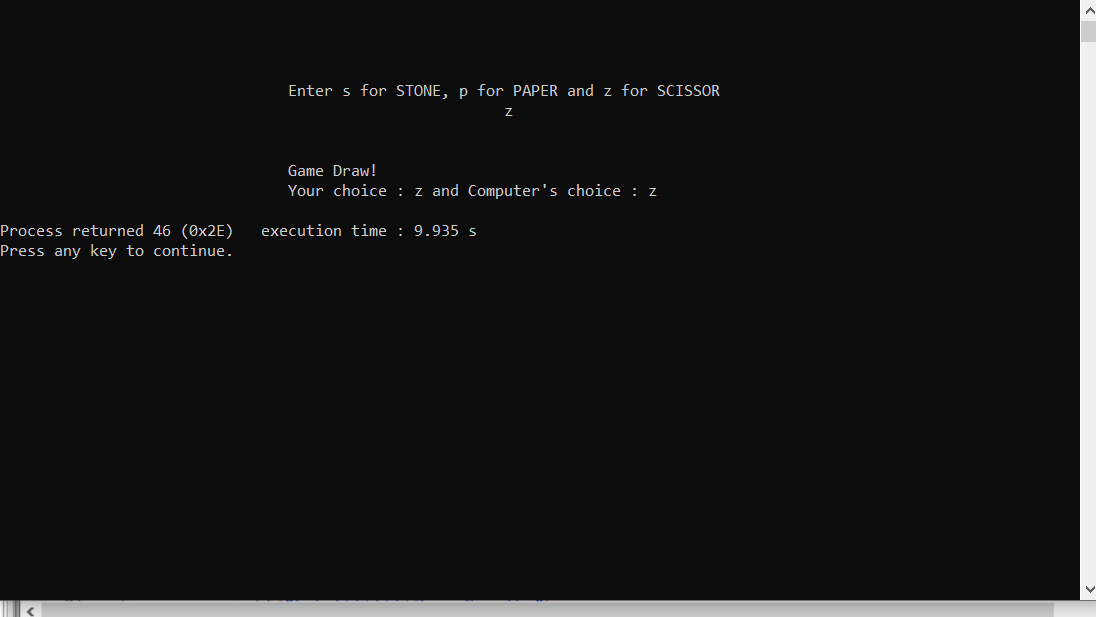Skip to content
Related Articles
Rock Paper Scissor in C
• Difficulty Level : Medium
• Last Updated : 12 Mar, 2021

Rock Paper Scissor (which is also called Stone Paper Scissor) is a hand game and played between two people, in which each player simultaneously forms one of three shapes. The winner of the game is decided as per the below rules:

• Rock vs Paper -> Paper wins.
• Rock vs Scissor -> Rock wins.
• Paper vs Scissor -> Scissor wins.

In this game, the user will be asked to make choice and according to the choice of user and computer and then the result will be displayed along with the choices of both computer and user.

Approach: Below is the functionality that needed to be implemented in the program:

• It consists of the declaration of the variables.
• printf() and scanf() functions for displaying the content and taking input from the user. It also contains  two predefined functions:
• srand() and rand() which are used to generate random numbers in the range [0, RAND_MAX) and srand() especially will help to generate a random number at each time.
• Take modulo of random number generated with 100 to make its range between (0 and 100).
• As the range is up to 100 only, the distribution among all the options i.e., stone, paper, and scissors is equal as all of them have an equal probability of coming.

Note: This random number will decide the choice of computer as:

• If the number is between 0-33 then the choice will be Stone.
• If the number is between 33-66 then the choice will be Paper.
• If the number is between 66-100 then the choice will be Scissors.

game() function: This function consists of if-else statements that will compare the choice of user and computer. If the user wins then it will return 1. Otherwise, if the computer wins then it will return 0. If it is a tie, it will return -1.

Below is the implementation of the above approach:

## C

 `// C program for the above approach``#include ``#include ``#include `` ` `// Function to implement the game``int` `game(``char` `you, ``char` `computer)``{``    ``// If both the user and computer``    ``// has chose the same thing``    ``if` `(you == computer)``        ``return` `-1;`` ` `    ``// If user's choice is stone and``    ``// computer's choice is paper``    ``if` `(you == ``'s'` `&& computer == ``'p'``)``        ``return` `0`` ` `            ``// If user's choice is paper and``            ``// computer's choice is stone``            ``else` `if` `(you == ``'p'` `&& computer == ``'s'``) ``return` `1;`` ` `    ``// If user's choice is stone and``    ``// computer's choice is scissor``    ``if` `(you == ``'s'` `&& computer == ``'z'``)``        ``return` `1;`` ` `    ``// If user's choice is scissor and``    ``// computer's choice is stone``    ``else` `if` `(you == ``'z'` `&& computer == ``'s'``)``        ``return` `0;`` ` `    ``// If user's choice is paper and``    ``// computer's choice is scissor``    ``if` `(you == ``'p'` `&& computer == ``'z'``)``        ``return` `0;`` ` `    ``// If user's choice is scissor and``    ``// computer's choice is paper``    ``else` `if` `(you == ``'z'` `&& computer == ``'p'``)``        ``return` `1;``}`` ` `// Driver Code``int` `main()``{``    ``// Stores the random number``    ``int` `n;`` ` `    ``char` `you, computer, result;`` ` `    ``// Chooses the random number``    ``// every time``    ``srand``(``time``(0));`` ` `    ``// Make the random number less``    ``// than 100, divided it by 100``    ``n = ``rand``() % 100;`` ` `    ``// Using simple probability 100 is``    ``// roughly divided among stone,``    ``// paper, and scissor``    ``if` `(n < 33)`` ` `        ``// s is denoting Stone``        ``computer = ``'s'``;`` ` `    ``else` `if` `(n > 33 && n < 66)`` ` `        ``// p is denoting Paper``        ``computer = ``'p'``;`` ` `    ``// z is denoting Scissor``    ``else``        ``computer = ``'z'``;`` ` `    ``printf``(``"\n\n\n\n\t\t\t\tEnter s "``           ``"for STONE, p for PAPER "``           ``"and z ``for` `SCISSOR\n\t\t\"``           ``"t\t\t\t\t"``);`` ` `    ``// input from the user``    ``scanf``(``"%c"``, &you);`` ` `    ``// Function Call to play the game``    ``result = game(you, computer);`` ` `    ``if` `(result == -1) {``        ``printf``(``"\n\n\t\t\t\tGame Draw!\n"``);``    ``}``    ``else` `if` `(result == 1) {``        ``printf``(``"\n\n\t\t\t\tWow! "``               ``"You have won the game!\n"``);``        ``else` `printf``(``"\n\n\t\t\t\tOh! You "``                    ``"have lost the game!\n"``);`` ` `        ``printf``(``"\t\t\t\tYOu choose : %c"``               ``" and Computer choose : "``               ``"%c\n"``,``               ``you, computer);`` ` `        ``return` `0;``    ``}`

Output:

• Firstly the user will be asked about the choice:• When the user enters the choice then the result is displayed:Want to learn from the best curated videos and practice problems, check out the C Foundation Course for Basic to Advanced C.

My Personal Notes arrow_drop_up